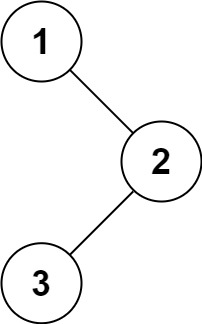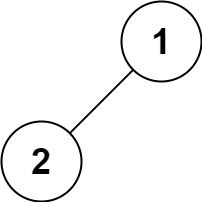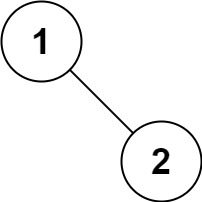# LeetCode: 144. Binary Tree Preorder Traversal

## 题目¶

Given the root of a binary tree, return the preorder traversal of its nodes' values.

Example 1:```Input: root = [1,null,2,3]
Output: [1,2,3]
```

Example 2:

```Input: root = []
Output: []
```

Example 3:

```Input: root = 
Output: 
```

Example 4:```Input: root = [1,2]
Output: [1,2]
```

Example 5:```Input: root = [1,null,2]
Output: [1,2]
```

Constraints:

• The number of nodes in the tree is in the range [0, 100].
• -100 <= Node.val <= 100

Follow up:

Recursive solution is trivial, could you do it iteratively?

## 解法¶

### 递归法实现前序遍历¶

```# Definition for a binary tree node.
# class TreeNode(object):
#     def __init__(self, val=0, left=None, right=None):
#         self.val = val
#         self.left = left
#         self.right = right
class Solution(object):
def preorderTraversal(self, root):
nodes = []
self._preorder(nodes, root)
return nodes

def _preorder(self, nodes, root):
if root is None:
return

nodes.append(root.val)
self._preorder(nodes, root.left)
self._preorder(nodes, root.right)
```

### stack 法实现前序遍历¶

```# Definition for a binary tree node.
# class TreeNode:
#     def __init__(self, val=0, left=None, right=None):
#         self.val = val
#         self.left = left
#         self.right = right
class Solution:
def preorderTraversal(self, root):
if root is None:
return []

nodes = []
stack = []
stack.append(root)
while stack:
curr = stack.pop()
nodes.append(curr.val)

# 因为 stack 是后进先出，所以要反着来
if curr.right is not None:
stack.append(curr.right)
if curr.left is not None:
stack.append(curr.left)

return nodes
```

## Comments

comments powered by Disqus